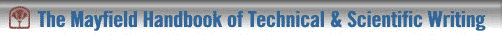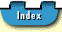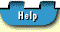## Comma Splice

Never link two independent clauses with just a comma; this is known as a comma splice error. You can correct a comma splice in four ways:

1. Separate the independent clauses into two separate sentences. Punctuate both sentences with periods.
2. Replace the comma with a semicolon or with a semicolon and a conjunctive adverb such as however or furthermore. (The conjunctive adverb is then normally followed by a comma.)
3. Replace the comma with a comma and a coordinating conjunction.
4. Make one of the clauses into a subordinate clause.

#### Unacceptable

```In 1931 Oppenheimer attempted to find an equation for the photon that would be an analogue to Dirac's equation for the electron, he failed in this effort.```

#### Acceptable

```In 1931 Oppenheimer attempted to find an equation for the photon that would be an analogue to Dirac's equation for the electron. He failed in this effort.```

#### Acceptable

```In 1931 Oppenheimer attempted to find an equation for the photon that would be an analogue to Dirac's equation for the electron; he failed in this effort.``` [or ". . . electron; however, he failed . . ."]

#### Acceptable

```In 1931 Oppenheimer attempted to find an equation for the photon that would be an analogue to Dirac's equation for the electron, but he failed in this effort.```

#### Acceptable

```Although in 1931 Oppenheimer attempted to find an equation for the photon that would be an analogue to Dirac's equation for the electron, he failed in this effort.```## Comma Splice ##